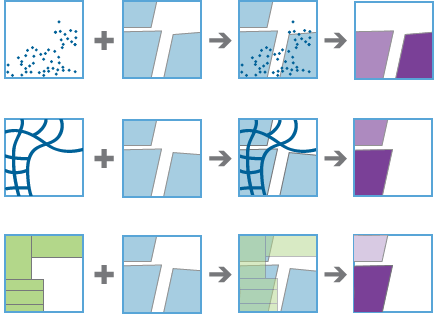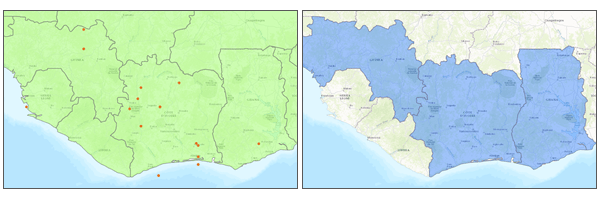# Summarize Within (GeoAnalytics Desktop)

## Summary

Overlays a polygon layer with another layer to summarize the number of points, length of the lines, or area of the polygons within each polygon and calculates attribute field statistics about those features within the polygons.

The following are example scenarios using Summarize Within:

• Given watershed boundaries and land-use boundaries by land-use type, calculate total acreage of land-use type for each watershed.
• Given county parcels and city boundaries, summarize the average value of vacant parcels within each city boundary.
• Given counties and roads, summarize the total mileage of roads by road type within each county.

## Illustration## Usage

• In simple terms, Summarize Within takes two layers, the input polygons and the input summary features, and stacks them on top of each other. After stacking these layers, you can look down through the stack and count the number of input summary features that fall within the input polygons. You can also calculate simple statistics about the attributes of the input summary features, such as sum, mean, minimum, maximum, and so on.

• Use Summarize Within to calculate standard statistics as well as geographically weighted statistics. Standard statistics summarize the statistical values without weighting. Weighted statistics calculate values using the geographically weighted values of the proportion of lines within a polygon, or the proportion of polygons within a polygon. Weighted statistics do not apply to points within polygons.

• You can calculate the lengths and areas of the summarized layers within each polygon using the options in the table below. Options are based on the geometry of the summarized layer.

Input featureDescriptionOption

Points

The count of summarized points within each polygon

None

Lines

The length of summarized lines within each polygon

• Miles
• Yards
• Feet
• Kilometers
• Meters

Areas

The area of summarized polygons within each polygon

• Square Miles
• Square Yards
• Square Feet
• Square Kilometers
• Square Meters
• Hectares
• Acres
• You can calculate standard statistics. For lines and areas, all weighted statistics will be calculated. Both the standard summary field statistics and the weighted summary field statistics are applied to data for the features in the Summarized Layer parameter that intersect the Summary Polygons layer. The weighted summary field statistics are multiplied by a weight based on the proportion of the features in the Summary Polygons parameter intersecting each feature in the Summarized Layer parameter.

• For standard statistics, there are eight options: count, sum, mean, minimum, maximum, range, standard deviation, and variance. There are two options for string statistics: count and any. There are eight weighted statistics that are calculated on numeric fields in the layer to be summarized: count, sum, mean, minimum, maximum, range, standard deviation, and variance.

• Weighted statistics are not calculated for string data. Each time Field and Statistic values are specified, a row is added to the tool pane so more than one statistic can be calculated. You can view the summarized results in the result layer's table or pop-ups. By default, the count of features intersecting the Summary Polygons values is always calculated.

• Analysis with binning requires that the input is projected or that the output coordinate system is set to a projected coordinate system. If the data is not in a projected coordinate system and you do not set one, a projection will be used based on the extent of the data you are analyzing.

• Optionally, a Group By Field value can be selected so statistics are calculated separately for each unique attribute value. When a Group By Field value is selected, a summary table listing each feature and statistic by Group By Field value is also created.

• The Add Minority and Majority Attributes and Add Group Percentages parameters are available when a Group By Field value is selected. The minority and majority will be the least and most dominant value from the Group By Field parameter, respectively, in which dominance is determined using the count of points, total length, or total area of each value.

• When the Add Minority and Majority Attributes parameter is checked, two fields will be added to the result layer. The fields will list the values from the Group By Field parameter that are the minority and majority for each result feature.

• The Add Group Percentages parameter is only available when Add Minority and Majority Attributes is checked. When the Add Group Percentages parameter is checked, two fields will be added to the result layer listing the percentage of the count of points, total length, or total area that belong to the minority and majority values for each feature. A percentage field will also be added to the result table listing the percentage of the count of points, total length, or total area that belong to all values from the Group By Field parameter for each feature.

• For weighted statistics, line layers are summarized using only the proportions of line features that are within the Summary Polygons value. Standard (nonweighted) statistics summarize any line intersecting the Summary Polygons value. When summarizing lines using weighted statistics, use counts and amounts (rather than rates or indices) so proportional calculations make logical sense in the analysis.

• Weighted statistics for summarized area layers are based on the proportions of the Summary Polygons features that are within the Summarized Layer value. When summarizing areas, use counts or amounts (rather than rates or indices) so proportional calculations make logical sense in the analysis.

• The output feature layer is always a polygon layer. Only polygons that intersect a summarized layer will be returned. Other polygons will be completely removed from the result layer.The input point and polygon features (the first image) and the resulting area features (the second image) are shown.

• The following fields are included in the output polygon features:

Field nameDescription
count

The count of summarized features that intersect each polygon layer.

sum_length_<linearunit>, or sum_area_<areaunit>

The total length of lines within the polygon or total area of summarized polygon within each polygon. These values are returned when Add shape summary attributes is checked and are returned in the specified unit.

statistic_<fieldname>

Specified statistics will each create an attribute field named in the following format: <statistic>_<fieldname>. For example, the maximum and standard deviation of the field id is MAX_id and SD_id.

pstatistic_<fieldname>

Specified weighted statistics will each create an attribute field named in the following format: p<statistic>_<fieldname>. For example, the weighted maximum of the field id is pMAX_id.

minority_<fieldname>

This value is returned when you create a group-by table and Add Minority and Majority Attributes is checked. This represents the values for the specified field that is the minority in each polygon. For example, there are five points in a polygon with a field called color and values of red, blue, blue, green, green. If you create a group by the color field, the value for the minority_color field is red.

majority_<fieldname>

This value is returned when you create a group-by table and Add Minority and Majority Attributes is checked. This represents the values for the specified field that is the majority in each polygon. For example, there are five points in a polygon with a field called color and values of red, blue, blue, green, green. If you create a group by the color field, the value for the minority_color field is blue;green.

minority_<fieldname>_percent

This value is returned when you create a group-by table and Add Group Percentages is checked. This represents the percentages of the count for the specified field that is the minority in each polygon. For example, there are five points in a polygon with a field called color and values of red, blue, blue, green, green. If you create a group by the color field, the value for the minority_color_percent field is 20 (calculated as 1/5).

majority_<fieldname>_percent

This value is returned when you create a group-by table and Add Group Percentages is checked. This represents the percentages of the count for the specified field that is the majority in each polygon. For example, there are five points in a polygon with a field called color and values of red, blue, blue, green, green. If you create a group by the color field, the value for the majority_color_percent field is 40 (calculated as 2/5).

join_id

This value is returned when you create a group-by table. This is an ID to link features to the group-by table. Every join_id field corresponds to one or more rows in the group-by table.

The following fields are included in the output group-by table:

Field nameDescription

join_id

This is an ID to link features to the polygon layer. Each polygon will have one or more features with the same ID that represent all of the group-by values. For example, there are five points in a polygon with a field called color and values of red, blue, blue, green, green. The group-by table will have three rows representing that polygon (same join ID), one for each color: red, blue, and green.

count

The count of the specified group within the joined polygon. For example, red is 1 for the selected polygon.

<statistic>_<fieldname>

Any specified statistic calculated for each group.

p<statistic>_<fieldname>

Any specified weighted statistic calculated for each group.

percentcount

The percentage each group contributes to the total count in the polygon. Using the example above, red contributes 1/5 = 20, blue contributes 2/5 = 40, and green contributes 2/5 = 20.

• You can improve the performance of the Summarize Within tool by doing one or more of the following:

• Set the extent environment so only data of interest will be analyzed.
• Larger bins will perform better than smaller bins. If you are using bins and are unsure which size to use, start with a larger bin to prototype.
• Use data that is local to where the analysis is being run.

• When running GeoAnalytics Desktop tools, the analysis is completed on your desktop machine. For optimal performance, data should be available on your desktop. If you are using a hosted feature layer, it is recommended that you use ArcGIS GeoAnalytics Server. If your data isn't local, it will take longer to run a tool. To use your ArcGIS GeoAnalytics Server to perform analysis, see GeoAnalytics Tools.

• Similar analysis can also be completed using the Summarize Within tool in the Standard Feature Analysis toolbox in ArcGIS Pro.

## Parameters

`arcpy.gapro.SummarizeWithin(summarized_layer, out_feature_class, polygon_or_bin, bin_type, {bin_size}, {summary_polygons}, sum_shape, {shape_units}, {standard_summary_fields}, {weighted_summary_fields}, {group_by_field}, {add_minority_majority}, {add_percentages}, {group_by_summary})`

### Code sample

SummarizeWithin example (Python window)

The following Python window script demonstrates how to use the SummarizeWithin function.

``````#-------------------------------------------------------------------------------
# Name: SummarizeWithin.py
# Description: Summarize river polylines by counties.

# Import system modules
import arcpy

arcpy.env.workspace = "C:/data/RedRiver_basin.gdb"

# Set local variables
summarizedLayer = "Rivers"
summaryPolys = "Basins"
summaryStatistics = [["Width", "MEAN"]]
weighedSummaryStatistics = [["DOC", "SUM"]]
out = 'SummarizedRivers'

# Execute SummarizeWithin
arcpy.gapro.SummarizeWithin(summarizedLayer, out, "POLYGON", None,
"KILOMETERS", summaryStatistics,
weightedSummaryStatistics)``````

• Basic: No
• Standard: No